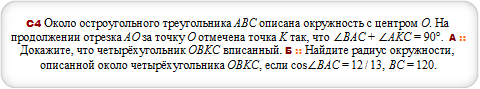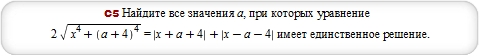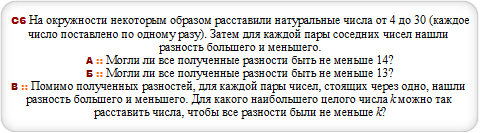# Закажи себе WolframAlpha|Pro! Получи пошаговые решения твоих задач!

## Досрочный ЕГЭ :: 28.04.2014 :: Вариант 4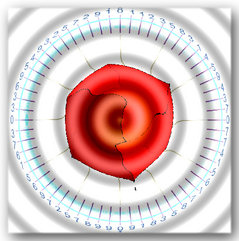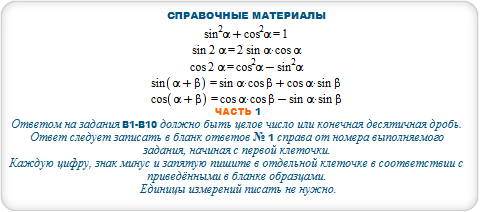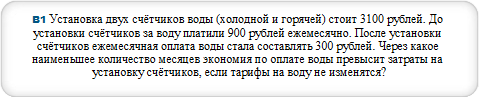#### ceil(a/(b-c)) where a = 3100, b = 900, c=300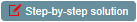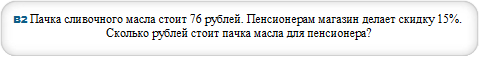#### b = N[a*(1-p/100)] where a=76,p=15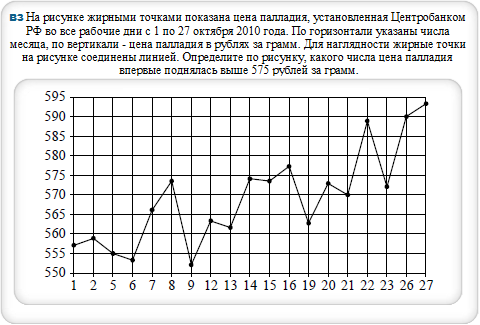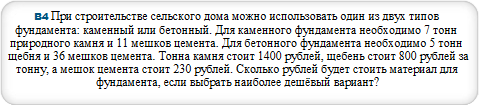#### B=m*v+n*w where m=5,n=36,v=800,w=230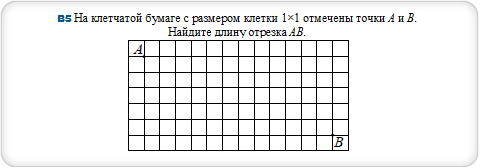#### c=sqrt(a^2+b^2) where a=12,b=5#### p=N[m/n] where m = 9, n = 45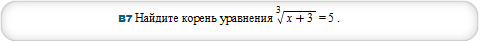#### solve (x+3)^(1/3)=5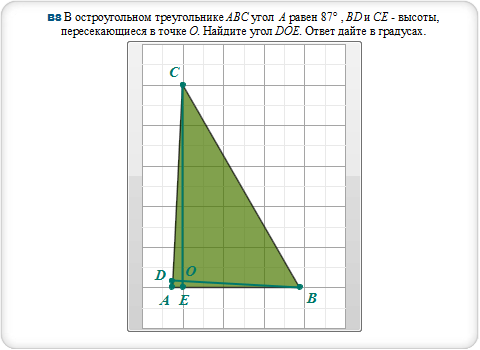#### 3D plot x^2+y^2+z^2=16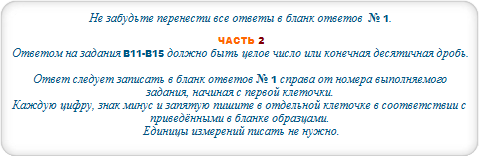#### T = (P/(sigma*S))^(1/4) where P = (228/100)*10^26, sigma = (57/10)*10^(-8), S = (1/64)*10^21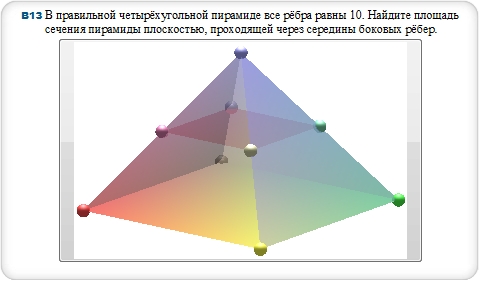#### s=(a/2)^2 where a=10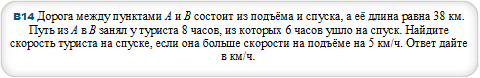#### v = (a*(T-t)+s)/T where s=38,T=8,t=6,a=5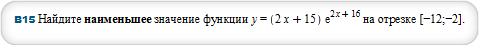#### 9^cos(x)+9^(-cos(x)) = 10/3, x from 2*Pi to 7*Pi/2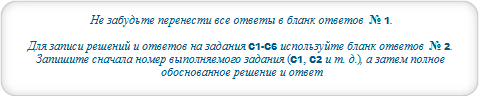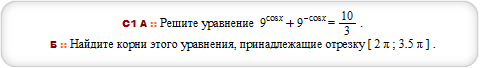#### 9^cos(x)+9^(-cos(x)) = 10/3, x from 2*Pi to 7*Pi/2#### solve {3^x+270/3^x >= 37,log(x-1,(x+3)/6)<= 0}